# InSAR Theory

## Producer Field Guide

HGD_Product
Producer Field Guide
HGD_Portfolio_Suite
Producer

Terrain height extraction is one of the most important applications for SAR images. There are two basic techniques for extracting height from SAR images: stereo and interferometry. Stereo height extraction is much like the optical process and is discussed in StereoSAR DEM Theory. The subject of this section is SAR interferometry (InSAR).

Height extraction from InSAR takes advantage of one of the unique qualities of SAR images: distance information from the sensor to the ground is recorded for every pixel in the SAR image. Unlike optical and IR images, which contain only the intensity of the energy received to the sensor, SAR images contain distance information in the form of phase. This distance is simply the number of wavelengths of the source radiation from the sensor to a given point on the ground. SAR sensors can record this information because, unlike optical and IR sensors, their radiation source is active and coherent.

Unfortunately, this distance phase information in a single SAR image is mixed with phase noise from the ground and other effects. For this reason, it is impossible to extract just the distance phase from the total phase in a single SAR image. However, if two SAR images are available that cover the same area from slightly different vantage points, the phase of one can be subtracted from the phase of the other to produce the distance difference of the two SAR images (hence the term interferometry). This is because the other phase effects for the two images are approximately equal and cancel out each other when subtracted. What is left is a measure of the distance difference from one image to the other. From this difference and the orbit information, the height of every pixel can be calculated.

This chapter covers basic concepts and processing steps needed to extract terrain height from a pair of interferometric SAR images.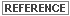For discussions of Single-Look Complex imagery, polarization, three types of imaging modes, and additional concepts, see the Radar Interferometry User Guide.

Electromagnetic Wave Background

In order to understand the SAR interferometric process, you must have a general understanding of electromagnetic waves and how they propagate. An electromagnetic wave is a changing electric field that produces a changing magnetic field that produces a changing electric field, and so on. As this process repeats, energy is propagated through empty space at the speed of light.

The figure below gives a description of the type of electromagnetic wave that we are interested in. In this diagram, E indicates the electric field and H represents the magnetic field. The directions of E and H are mutually perpendicular everywhere. In a uniform plane, wave E and H lie in a plane and have the same value everywhere in that plane.

A wave of this type with both E and H transverse to the direction of propagation is called a Transverse ElectroMagnetic (TEM) wave. If the electric field E has only a component in the y direction and the magnetic field H has only a component in the z direction, then the wave is said to be polarized in the y direction (vertically polarized). Polarization is generally defined as the direction of the electric field component with the understanding that the magnetic field is perpendicular to it.

Electromagnetic Wave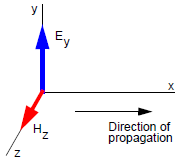The electromagnetic wave described above is the type that is sent and received by an SAR. The SAR, like most equipment that uses electromagnetic waves, is only sensitive to the electric field component of the wave; therefore, we restrict our discussion to it. The electric field of the wave has two main properties that we must understand in order to understand SAR and interferometry. These are the magnitude and phase of the wave. The figure below shows that the electric field varies with time.

Variation of Electric Field in Time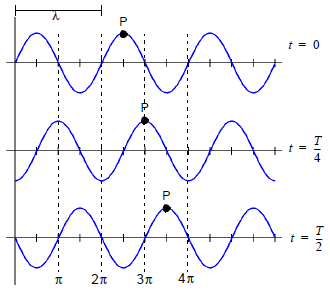The figure shows how the wave phase varies with time at three different moments. In the figure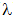is the wavelength and T is the time required for the wave to travel one full wavelength. P is a point of constant phase and moves to the right as time progresses. The wave has a specific phase value at any given moment in time and at a specific point along its direction of travel. The wave can be expressed in the form of Equation 1.

Equation 1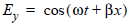Where: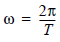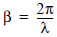Equation 1 is expressed in Cartesian coordinates and assumes that the maximum magnitude of Ey is unity. It is more useful to express this equation in exponential form and include a maximum term as in Equation 2.

Equation 2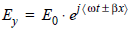So far we have described the definition and behavior of the electromagnetic wave phase as a function of time and distance. It is also important to understand how the strength or magnitude behaves with time and distance from the transmitter. As the wave moves away from the transmitter, its total energy stays the same but is spread over a larger distance. This means that the energy at any one point (or its energy density) decreases with time and distance as shown in the following figure.

Effect of Time and Distance on Energy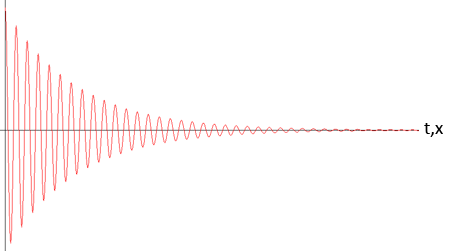The magnitude of the wave decreases exponentially as the distance from the transmitter increases. Equation 2 represents the general form of the electromagnetic wave that we are interested in for SAR and InSAR applications. Later, we further simplify this expression given certain restrictions of an SAR sensor.

Interferometric Model

Most uses of SAR imagery involve a display of the magnitude of the image reflectivity and discard the phase when the complex image is magnitude-detected. The phase of an image pixel representing a single scatterer is deterministic; however, the phase of an image pixel represents multiple scatterers (in the same resolution cell), and is made up of both a deterministic and nondeterministic, statistical part. For this reason, pixel phase in a single SAR image is generally not useful. However, with proper selection of an imaging geometry, two SAR images can be collected that have nearly identical nondeterministic phase components. These two SAR images can be subtracted, leaving only a useful deterministic phase difference of the two images.

The following figure provides the basic geometric model for an interferometric SAR system.

Geometric Model for an Interferometric SAR System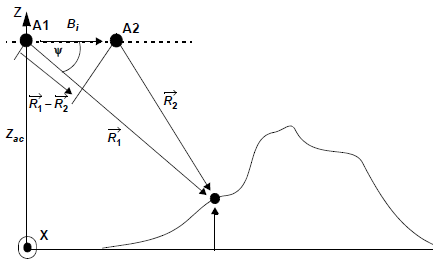Where:

A1 = antenna 1

A2 = antenna 2

Bi = baseline

R1 = vector from antenna 1 to point of interest

R2 = vector from antenna 2 to point of interest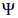= depression angle between R1 and baseline vectors (= 90° - look angle)

Zac = antenna 1 height

A rigid baseline Bi separates two antennas, A1 and A2. This separation causes the two antennas to illuminate the scene at slightly different depression angles relative to the baseline. Here, Y is the nominal depression angle from A1 to the scatterer relative to the baseline. The model assumes that the platform travels at constant velocity in the X direction while the baseline remains parallel to the Y axis at a constant height Zac above the XY plane.

The electromagnetic wave defined in Equation 2 describes the signal data collected by each antenna. The two sets of signal data differ primarily because of the small differences in the data collection geometry. Complex images are generated from the signal data received by each antenna.

As stated earlier, the phase of an image pixel represents the phase of multiple scatters in the same resolution cell and consists of both deterministic and unknown random components. A data collection for SAR interferometry adheres to special conditions to ensure that the random component of the phase is nearly identical in the two images. The deterministic phase in a single image is due to the two-way propagation path between the associated antenna and the target.

From our previously derived equation for an electromagnetic wave, and assuming the standard SAR configuration in which the perpendicular distance from the SAR to the target does not change, we can write the complex quantities representing a corresponding pair of image pixels, P1 and P2, from image 1 and image 2 as Equation 3 and Equation 4.

Equation 3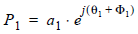and

Equation 4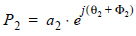The quantities a1 and a2 represent the magnitudes of each image pixel. Generally, these magnitudes are approximately equal. The quantities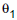and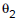are the random components of pixel phase. They represent the vector summations of returns from all unresolved scatterers within the resolution cell and include contributions from receiver noise. With proper system design and collection geometry, they are nearly equal. The quantities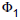and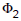are the deterministic contribution to the phase of the image pixel. The desired function of the interferometer is to provide a measure of the phase difference,-.

Next, we must relate the phase value to the distance vector from each antenna to the point of interest. This is done by recognizing that phase and the wavelength of the electromagnetic wave represent distance in number of wavelengths. Equation 5 relates phase to distance and wavelength.

Equation 5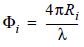Multiplication of one image and the complex conjugate of the second image on a pixel-by-pixel basis yields the phase difference between corresponding pixels in the two images. This complex product produces the interferogram I with:

Equation 6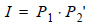Where ' denotes the complex conjugate operation. Withandnearly equal and a1 and a2 nearly equal, the two images differ primarily in how the slight difference in collection depression angles affectsand. Ideally then, each pixel in the interferogram has the form:

Equation 7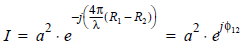using a1 = a2 = a. The amplitude a2 of the interferogram corresponds to image intensity. The phase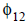of the interferogram becomes

Equation 8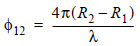which is the quantity used to derive the depression angle to the point of interest relative to the baseline and, eventually, information about the scatterer height relative to the XY plane. Using the following approximation allows us to arrive at an equation relating the interferogram phase to the nominal depression angle.

Equation 9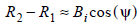Equation 10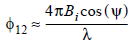In Equation 9 and Equation 10,is the nominal depression angle from the center of the baseline to the scatterer relative to the baseline. No phase difference indicates that= 90 degrees and the scatterer is in the plane through the center of and orthogonal to the baseline. The interferometric phase involves many radians of phase for scatterers at other depression angles since the range difference R2 - R1 is many wavelengths. In practice, however, an interferometric system does not measure the total pixel phase difference. Rather, it measures only the phase difference that remains after subtracting all full 2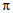intervals present (modulo-2).

To estimate the actual depression angle to a particular scatterer, the interferometer must measure the total pixel phase difference of many cycles. This information is available, for instance, by unwrapping the raw interferometric phase measurements beginning at a known scene location.Phase unwrapping is discussed in further detail in Phase Unwrapping later in this section.

Because of the ambiguity imposed by the wrapped phase problem, it is necessary to seek the relative depression angle and relative height among scatterers within a scene rather then their absolute depression angle and height. The differential of Equation 10 with respect toprovides this relative measure. This differential is

Equation 11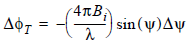or

Equation 12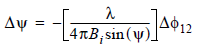This result indicates that two pixels in the interferogram that differ in phase byrepresent scatterers differing in depression angle by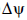. The figure below shows the differential collection geometry.

Differential Collection Geometry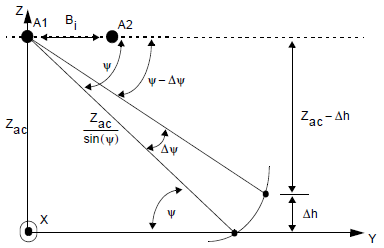From this geometry, a changein depression angle is related to a change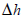in height (at the same range from mid-baseline) by Equation 13.

Equation 13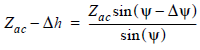Using a useful small-angle approximation to Equation 13 and substituting Equation 12 into Equation 13 provides the result Equation 14 for.

Equation 14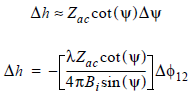Note that, because we are calculating differential height, we need at least one known height value in order to calculate absolute height. This translates into a need for at least one GCP in order to calculate absolute heights from InSAR process.

In this section, we have derived the mathematical model needed to calculate height from interferometric phase information. In order to put this model into practice, there are several important processes that must be performed. These processes are image coregistration, phase noise reduction, phase flattening, and phase unwrapping. These processes are discussed in the following sections.

Image Coregistration

In the discussion of the interferometric model of the last section, we assumed that the pixels had been identified in each image that contained the phase information for the scatterer of interest. Aligning the images from the two antennas is the purpose of the image coregistration step. For interferometric systems that employ two antennas attached by a fixed boom and collect data simultaneously, this coregistration is simple and deterministic. Given the collection geometry, the coregistration can be calculated without referring to the data. For repeat pass systems, the coregistration is not quite so simple. Since the collection geometry cannot be precisely known, we must use the data to help us achieve image coregistration.

Coregistration model is based on a first order polynomial:

match_sample =
coefs_xx + coefs_xx × ref_sample + coefs_xx × ref_line

match_line =
coefs_xy + coefs_xy × ref_sample + coefs_xy × ref_line

Where:

(ref_sample, ref_line) = Reference SAR image's coordinates in range and azimuth direction respectively.

(match_sample, match_line) = Corresponding match SAR image's coordinates in range and azimuth direction respectively after coregistration.

coefs_xx = Array of length 3 representing polynomial coefficients, which describe the subpixel shift of match image along Range (x-direction).

coefs_xy = Array of length 3 representing polynomial coefficients, which describe the subpixel shift of match image along Azimuth (y-direction).

The coregistration process for repeat pass interferometric systems is generally broken into two steps: pixel and sub-pixel coregistration. Pixel coregistration involves using the magnitude (visible) part of each image to remove the image misregistration down to around a pixel. This means that, after pixel coregistration, the two images are coregistered to within one or two pixels of each other in both the range and azimuth directions.

Pixel coregistration is best accomplished using a standard window correlator to compare the magnitudes of the two images over a specified window. You usually specify a starting point in the two images, a window size, and a search range for the correlator to search over. The process identifies the pixel offset that produces the highest match between the two images, and therefore the best interferogram. One offset is enough to pixel coregister the two images.

Pixel coregistration, in general, produces a reasonable interferogram, but not the best possible. This is because of the nature of the phase function for each of the images. In order to form an image from the original signal data collected for each image, it is required that the phase functions in range and azimuth be Nyquist sampled.

Nyquist sampling simply means that the original continuous function can be reconstructed from the sampled data. This means that, while the magnitude resolution is limited to the pixel sizes (often less than that), the phase function can be reconstructed to much higher resolutions. Because it is the phase functions that ultimately provide the height information, it is important to coregister them as closely as possible. This fine coregistration of the phase functions is the goal of the sub-pixel coregistration step.

Sub-pixel coregistration is achieved by starting at the pixel coregistration offset and searching over upsampled versions of the phase functions for the best possible interferogram. When this best interferogram is found, the sub-pixel offset has been identified. In order to accomplish this, we must construct higher resolution phase functions from the data. In general this is done using the relation from signal processing theory shown in Equation 15.

Equation 15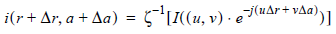Where:

r = range independent variable

a = azimuth independent variable

i(r, a) = interferogram in spacial domain

I(u, v) = interferogram in frequency domain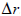= sub-pixel range offset (that is, 0.25)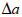= sub-pixel azimuth offset (that is, 0.75)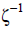= inverse Fourier transform

Applying this relation directly requires two-dimensional (2D) Fourier transforms and inverse Fourier transforms for each window tested. This is impractical given the computing requirements of Fourier transforms. Fortunately, we can achieve the upsampled phase functions we need using 2D raised cosine interpolation, which involves convolving a 2D raised cosine function of a given size over our search region. Equation 16 defines the raised cosine function for one dimension.

Equation 16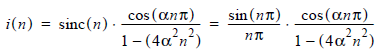Where: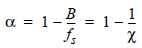in which:

B = system bandwidth in megahertz, for example, ERS 15.5 MHz

fs = sampling frequency in megahertz, for example, ERS 18.96 MHz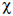= oversampling ratio, for example, 1.223 (possible values are in the range of [1.0,1.4] with a step size of 0.01)

Using raised cosine interpolation is a fast and efficient method of reconstructing parts of the phase functions which are at sub-pixel locations.

In general, one sub-pixel offset is not enough to sub-pixel coregister two SAR images over the entire collection. Unlike the pixel coregistration, sub-pixel coregistration is dependent on the pixel location, especially the range location. For this reason, it is important to generate a sub-pixel offset function that varies with range position. Two sub-pixel offsets, one at the near range and one at the far range, are enough to generate this function. This sub-pixel coregister function provides the weights for the raised cosine interpolator needed to coregister one image to the other during the formation of the interferogram.

Phase Noise Reduction

In Interferometric Model earlier in this section, note that it is necessary to unwrap the phase of the interferogram before it can be used to calculate heights. From a practical and implementational point of view, the phase unwrapping step is the most difficult.See discussion of phase unwrapping in Phase Unwrapping, several sections below.

Before unwrapping, we can do a few things to the data that make phase unwrapping easier. First, reduce the noise in the interferometric phase function. Phase noise is introduced by radar system noise, image misregistration, and speckle effects caused by the complex nature of the imagery. Reducing this noise is done by applying a coherent average filter of a given window size over the entire interferogram. This filter is similar to the more familiar averaging filter, except that it operates on the complex function instead of just the magnitudes. The form of this filter is given in Equation 17.

Equation 17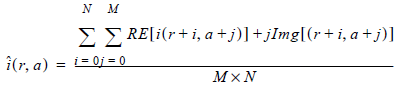The "Interferometric Phase Image without Filtering" figure shows an interferometric phase image without filtering.

The "Interferometric Phase Image with Filtering" shows the same phase image with filtering.

Interferometric Phase Image without Filtering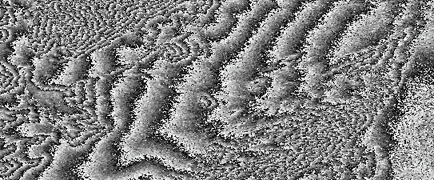Interferometric Phase Image with Filtering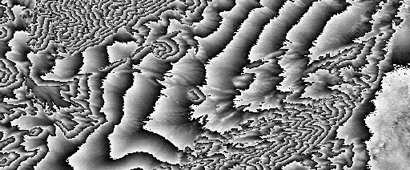The sharp ridges that look like contour lines in the two figures above show where the phase functions wrap. The goal of phase unwrapping step is to make this one continuous function.

Notice how the filtered image of the second figure above is much cleaner than that of the first figure above. This filtering makes phase unwrapping much easier.

Phase Flattening

The phase function of the "Interferometric Phase Image with Filtering" figure above is fairly well behaved and is ready to be unwrapped. There are relatively few wrap lines and they are distinct. Notice in the areas where the elevation is changing more rapidly (mountain regions) the frequency of the wrapping increases. In general, the higher the wrapping frequency, the more difficult the area is to unwrap. Once the wrapping frequency exceeds the spacial sampling of the phase image, information is lost. An important technique in reducing this wrapping frequency is phase flattening.

Phase flattening involves removing high frequency phase wrapping caused by the collection geometry. This high frequency wrapping is mainly in the range direction, and is because of the range separation of the antennas during the collection. Recall that it is this range separation that gives the phase difference and therefore the height information. The phase function of the "Interferometric Phase Image with Filtering" figure above has already had phase flattening applied to it. The following figure shows this same phase function without phase flattening applied.

Interferometric Phase Image without Phase Flattening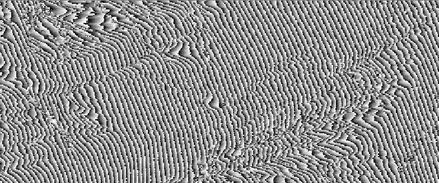Phase flattening is achieved by removing the phase function that would result if the imaging area was flat from the actual phase function recorded in the interferogram. It is possible, using the equations derived in Interferometric Model, described earlier in this section, to calculate this flat Earth phase function and subtract it from the data phase function.

It should be obvious that the phase function in the "Interferometric Phase Image with Filtering" figure is easier to unwrap then the phase function of the :Interferometric Phase Image without Phase Flattening" figure.

Phase Unwrapping

We stated in Interferometric Model, described earlier in this section, that we must unwrap the interferometric phase before we can use it to calculate height values. In Phase Noise Reduction and Phase Flattening, both described earlier in this section, we develop methods of making the phase unwrapping job easier. This section further defines the phase unwrapping problem and describes how to solve it.

As an electromagnetic wave travels through space, it cycles through its maximum and minimum phase values many times as shown in the following figure..

Electromagnetic Wave Traveling through Space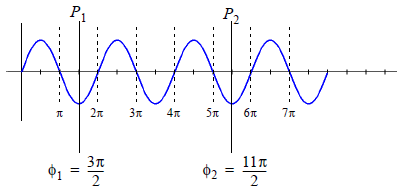The phase difference between points P1 and P2 is given by Equation 18.

Equation 18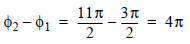Recall from Equation 8 that finding the phase difference at two points is the key to extracting height from interferometric phase. Unfortunately, an interferometric system does not measure the total pixel phase difference. Rather, it measures only the phase difference that remains after subtracting all full 2intervals present (modulo 2). This results in the following value for the phase difference of Equation 18.

Equation 19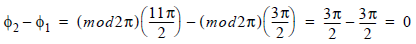The following figure further illustrates the difference between a one-dimensional continuous and wrapped phase function. Notice that when the phase value of the continuous function reaches 2, the wrapped phase function returns to 0 and continues from there. The job of the phase unwrapping is to take a wrapped phase function and reconstruct the continuous function from it.

One-dimensional Continuous vs. Wrapped Phase Function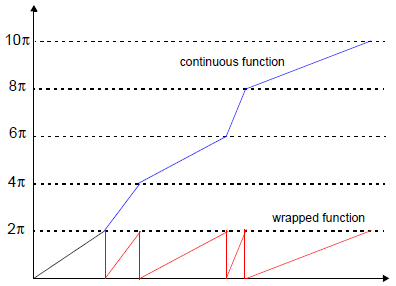There has been much research and many different methods derived for unwrapping the 2D phase function of an interferometric SAR phase image. A detailed discussion of all or any one of these methods is beyond the scope of this chapter. The most successful approaches employ algorithms which unwrap the easy or good areas first and then move on to more difficult areas. Good areas are regions in which the phase function is relatively flat and the correlation is high. This prevents errors in the tough areas from corrupting good regions. The figure below shows a sequence of unwrapped phase images for the phase function of the "Interferometric Phase Image with Filtering" figure, shown earlier in this section.

Sequence of Unwrapped Phase Images